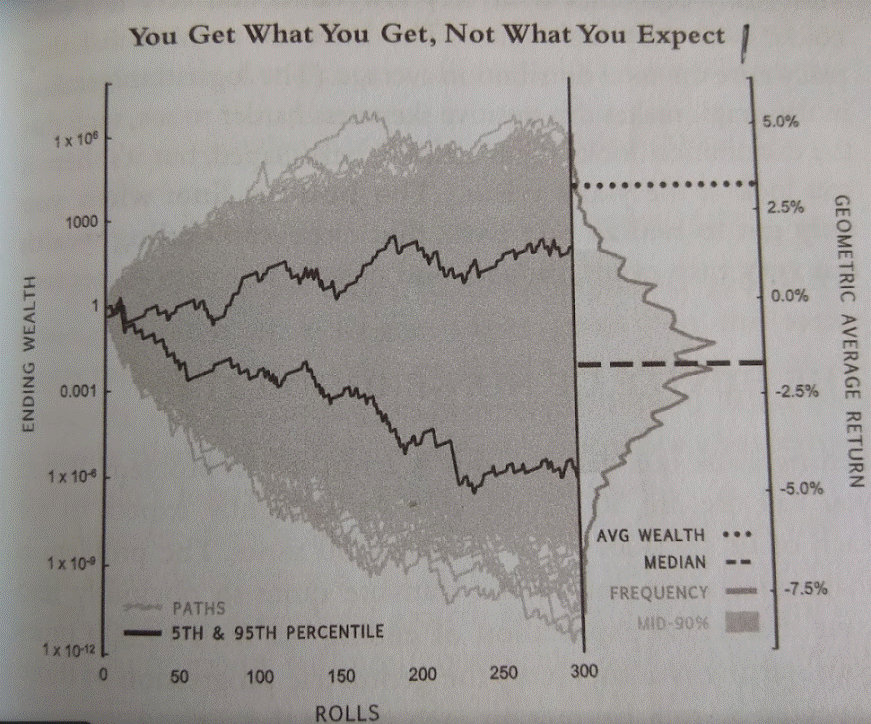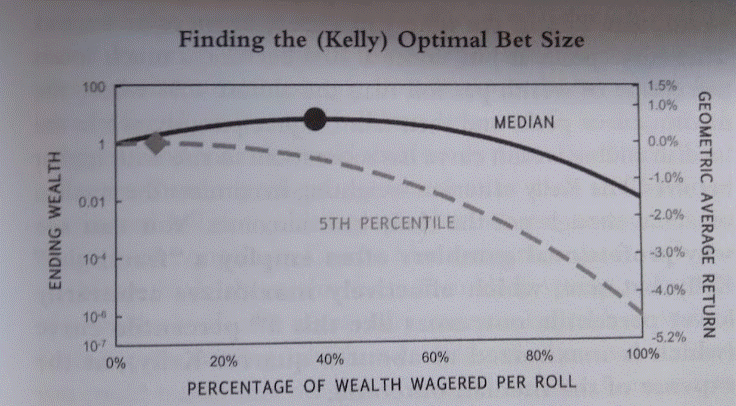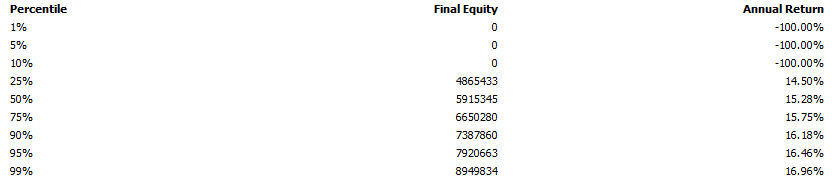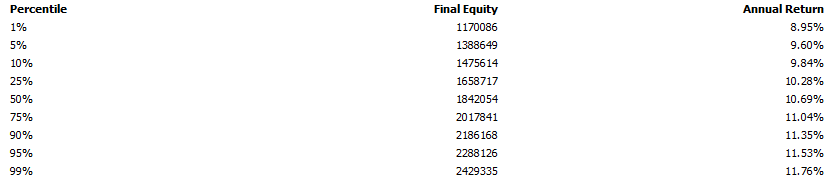# Why Arithmetic And Geometric Averages Differ In Trading And Investing (Position Sizing And The Kelly Criterion)

Last Updated on November 12, 2021 by Oddmund Groette

Albert Einstein is famous for saying that compounding is the eighth wonder. But what if he is wrong? Perhaps multiplicative compounding is the most destructive force in the universe? The sequence of returns and the different alternative paths ultimately determine your geometrical average which might be far away from the arithmetic average. Don’t be fooled by the arithmetic average.

The arithmetic and geometric averages/means and returns differ in trading and investing because the arithmetic average is mainly a theoretical average, while the geometric average takes into account the sequence of returns (or paths) of an investment. The arithmetic average might be positive, but you can still end up with losses – even ruin. The reason is the volatility tax. The multiplicative effects of compounding might leave you with losses you never recover from. Your sequence of returns is dependant on your position sizing. Thus, we end the article by explaining the Kelly Criterion which is all about finding the optimal position/betting sizing.

If your strategy has a positive expected average gain per trade, the end result still might be catastrophic. The reason is due to path/sequence and compound average growth rate (CAGR). CAGR, the geometric return, mean, or average, is the correct measurement and is different from the arithmetic return.

## What is the arithmetic average / arithmetic mean / arithmetic return?

Let’s assume you have a strategy that returns the following sequence of ten trades measured in percentage: 11, 33, 6, -5, 7, 21, -19, -9, 29, and -24. If you add all the numbers and divide by the number of observations (10), you get 5%. This means that the average gain per trade was 5%. This is the arithmetic average.

The difference between your starting capital and how much you end up with is not the arithmetic return, but the CAGR or geometric average / geometric mean:

## What is the geometric average / geometric mean / geometric return?

Now, if you start with 100 000 and add or deduct the above ten trades, you end up with 139 092. This equals a geometric return (CAGR) of 3.35%, much lower than the arithmetic average of 5%.

The compound annual growth rate (CAGR) is the return on an investment over a certain period of time. This is why it differs from the arithmetic average. It takes into account the compounding from the start to the finish. This means that the arithmetic and geometric average are two completely different things.

## Why do the arithmetic and geometrical average / mean / return differ?

They differ because the arithmetic average is mainly a theoretical value while the geometrical average is what you get in real life based on the sequence of returns. Mark Spitznagel writes in his Safe Haven book: you get what you get, not what you expect.

If you roll the dice you might not get the theoretical average because of the sequence and order of the rolling of the dice. You might get 1, 4, and 3 when you roll, while the next sequence might be 2, 6, and 1. Depending on the expected gain on each number of the dice, the end result fluctuates wildly. We show this by an example further down.

In the example above we got a lower CAGR and geometric average than the arithmetic average. Why do we get worse results when using the geometric return? It’s because of the volatility tax:

## What is the volatility tax?

Over my 25yrs in the game of quantitative investing, and drenched in equations and models, I’ve honed in on the only math that really matters: Minimize drawdowns during unfavorable times. The multiplicative nature of positive returns during good times does all the rest.

The name volatility tax is taken from Mark Spitznagel’s book called Safe Haven – Investing For Financial Storms. Spitznagel defines the volatility tax like this:

It’s a tax extracted by the multiplicative dynamics of compounding, what I have dubbed the volatility tax.

The volatility tax is a tax well hidden in the arithmetic average. The geometric average is lower because you suffer drawdowns that are hard to recover from. If you have a 33% loss in one year, you need a 49% return to get back to even. If you lose 50%, you need to get 100% to recoup.This is why Spitznagel calls this a volatility tax. Just ONE devastating loss might not lower the arithmetic average significantly, but it can lower the geometric average significantly – even lead to ruin! This is why you need to control your losses and control your position sizing.

## An example of arithmetic vs geometric average / geometric mean / geometric return

Mark Spitznagel has a very illustrative example in his book about Safe Haven Investing. He made up a gamble of rolling dice with the following three different outcomes:

• If 1 comes up, you lose 50% of your equity.
• If 2, 3, 4, and 5 come up, you gain 5% of your equity.
• If 6 comes up, you gain 50% of your equity.

The arithmetic return of the game is 3.3%: (-50, 5, 5, 5, 5, 50)/6.

This means your expected gain per roll is 3.3%. If you start with 1 dollar and roll the dice 300 times, you end up with 18 713 (1.033 x 1.033 x 1.033….. 300 times). Compounding the 3.3% gain per roll leaves you with almost 19 000 times your starting wealth, on average. Not bad!

However, before you buy champagne and order escorts you really need to think things through. The problem is that when you roll the dice you will not get the arithmetic average. You only get to walk one path. You better aim for survival!

In fact, you will never get the arithmetic average because it’s just a theoretical number. You either lose 50%, gain 5%, or gain 50%. That is the only possible outcome of each roll of the dice. An arithmetic average is just a theoretical number that exists on paper. This is the difference between being street smart vs. book smart.

The huge difference between each roll of the dice implies you face the risk of an unlucky roll – something called sampling error. On average one in six rolls will cut your equity in half! You might take comfort in that this is a fair game and if you keep rolling the dice your expected gain will regress to the mean of 3.3%.

But wait a minute. Let’s test if the latter reasoning is correct:

You start rolling the dice and the first six rolls return 3, 6, 1, 5, 4, and 2. What is your equity after those six rolls?

1 (starting capital) x 1.05 x 1.5 x 0.5 x 1.05 x 1.05 x 1.05 = 0.912

Each side of the dice turned up once and your equity is still less than what you started with. Shouldn’t you get the average 3.3% gain when all sides of the dice turned up once?

The problem in the real world is that you only get one path or sequence – not all of the 300 rolls. As Spitznagel writes in his book:

You get what you get, not what you expect!

What happens if you play out different paths ten thousand separate times (each with 300 rolls of the dice)?

The illustration below from Mark Spitznagels Safe Haven Investing shows the frequency distribution (read more about Monte Carlo simulation in trading and investing):In real life, you can only traverse only one of these ten thousand paths. You better pick the right one!

Even though you can expect each side to turn up equally on all sides about 50 times in a 300 roll (one path), the arithmetic average doesn’t translate into the same real-life geometric average:

If each side of the dice comes up an equal 50 times (300/6) on each side, your entire equity is almost wiped out:

0.5^50 x 1.05^50 x 1.05^50 x 1.05^50 x 1.05^50 x 1.5^50 = 0.01

You risk losing 99% of your life savings! Mark Spitznagel calls multiplicative compounding the most destructive force in the universe. Perhaps Einstein was wrong in labeling compounding as the eighth wonder?

What is the geometric average of sampling ten thousand paths of 300 rolls?

Unfortunately, the geometric average is a negative 1.5%: 0.01^(1/300). Your 3.3% positive arithmetic average will most likely end up with a loss in the real world.

Most retail investors perform much worse than the benchmarks, and one reason for that is big losses that multiplicates. If you “choose” the wrong path, you are unlikely to ever recover. You are lost.

This is why you should always trade with a smaller size than you’d like.

What is the optimal betting size? Let’s look at the Kelly Criterion:

## What is the Kelly Criterion?

Based on the difference between the arithmetic and geometric averages a mathematician named John Kelly made a formula in 1956 for the optimal betting size when the expected returns are known. The criterion is based on the expected geometric return and not the arithmetic average.

The Kelly Criterion is based on the logarithmic scale of the geometric expected return. It maximizes the expected value by considering the risk of ruin and losses.

#### How to calculate the optimal betting size by using the Kelly Criterion

The Kelly Criterion is straightforward to calculate: you only need two inputs to determine the optimal betting size:

1. The win/loss ratio – the win percentage of your trading strategy (R) (dividing the total gains of the winning trades by the total loss of the losing trades)
2. The win ratio of the trading strategy (W) (the number of trades that showed a profit divided by the number of trades that made a loss)

These two variables you need to put into this formula:

Kelly % = W – [(1 – W) / R]

Let’s assume you have a strategy you want to trade after a successful out-of-sample backtest. The backtest had these numbers:

• Total gains of the winning trades: 9 229 137
• Total loss of the losing trades: 3 206 730
• (The win/loss ratio is thus 2.88)
• The win ratio is 74% (thus 26% of the trades showed a loss)

Putting this into the formula above yields this result:

Kelly % = 0.74 – [(1 – 0.74) / 2.88]  = 0.6497

Thus, the optimal betting size according to the Kelly formula is 0.65 of your equity.

Of course, we don’t know the future outcome of a trading strategy so the Kelly Criterion should be used carefully. Also, keep in the back of your mind that the biggest drawdown is yet to come. As we always emphasize, always trade a smaller size than you’d like.

## Why betting size is extremely important

Instead of betting 100% of your equity on each roll, you bet only 40% of your equity while the remaining 60% sits idle and yields nothing. This means your arithmetic average drops to 1.32%.

But a funny thing happens to your expected end result: the geometric average improves from a negative 1.5% to a positive 0.6%!

Mark Spitznagel has the following diagram which shows the expected geometric average return for the different betting sizes:There is even a more famous and illustrative betting game that illustrates the importance of the betting size:

#### The optimal betting size in a coin toss

In a behavioral study done by scientists, a group of 61 people was given 25 USD and asked to place even money on a game that would land heads 60% of the time. There was a time limit of 30 minutes and the coin was tossed 10 times a minute, hence the participants could place a maximum of 300 bets. The prizes were capped at 250 USD.

What is the optimal betting size? According to the Kelly Criterion, the optimal betting size is about 20% which equals about 2% gain per toss. This equals a maximum potential gain of 10 500 USD if it wasn’t for the capped 250 USD winning size.

How did the participants perform in this game with a highly positive expectancy? It turns out 28% went bust and the average payout was just 91 USD. The max limit was reached by only 21% of the participants.

Furthermore, 18% of the 61 participants bet everything on one toss, and even 67% of the group at one point bet on tails at least once. If everyone had followed the betting size of the Kelly Criterion, about 95% of the players would have reached the limit of max profits.

Below is the backtest report of a trading strategy in the S&P 500. The backtest is from SPY’s inception in 1993 until October 2021. The backtest had the following statistics:

• CAGR 15.4% if 100% equity allocation (buy and hold was 10.4%)
• The average gain per trade was 0.8%
• The win ratio was 74%
• The average winner was 1.68%
• The average loser was a negative 1.72%
• The profit factor was a solid 2.88
• The Sharpe Ratio was 2

If we allocate 100% of our equity on each trade for this strategy, Amibroker gave the following results for a simulated Monte Carlo analysis:Even with such good backtest statistics, the strategy has a pretty high chance of going bust (10%).

If we lower the allocation to 70% of the equity, the optional allocation is between 65 and 70%, we get the following numbers:The annual return is lower for all simulations because we allocate less capital, but we have a zero chance of going bust. As Nassim Nicholas Taleb often reminds us, first we must survive. All else is secondary.

## Conclusion about arithmetic and geometric averages:

Don’t be fooled by the arithmetic average. The sequence of returns might lead to completely different geometrical returns and averages.

In real life, you can only traverse one path, and you better make sure you have a margin of safety in case that path turns out to be wrong. You get only one path to survival, you don’t get the average of paths. Multiplicative compounding is fantastic if you manage to get on the right track, but detrimental if you get it wrong.

Even with a positive expectancy, you can highly likely suffer losses – even ruin – if you don’t have the optimal position size. We end the article by yet again emphasizing our main trading rule:

Always trade smaller than you’d like.

Disclaimer: We are not financial advisors. Please do your own due diligence and investment research or consult a financial professional. All articles are our opinions – they are not suggestions to buy or sell any securities.